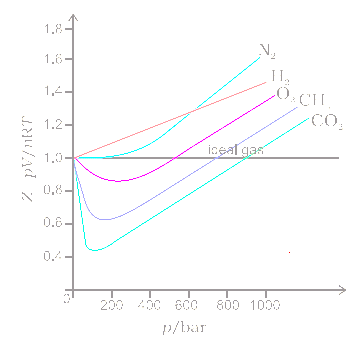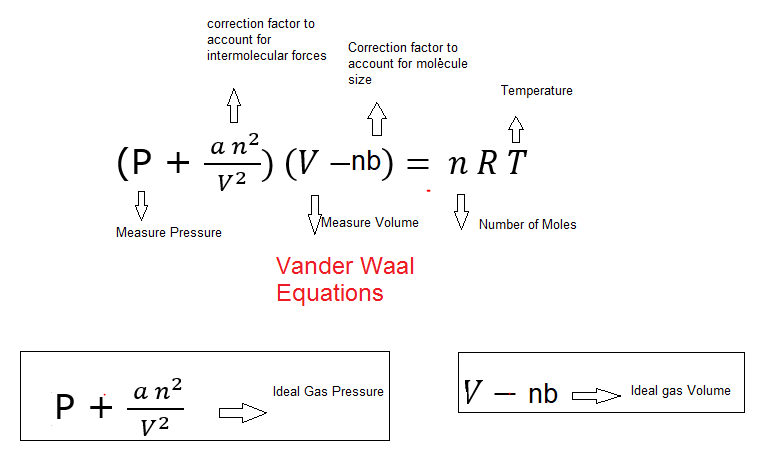# Van der waals equation

Table of Content

## Deviation from Ideal Gas Behaviour

Real Gases: -
• A gas which do not follow ideal gas behaviour under all conditions of temp. and pressure is called real gas.
• Deviation from ideal behaviour with respect to pressure can be studied by plotting pressure versus volume curve at constant temp. (Boyle’s law)

Compressibility factor (z): -
$z =\frac {PV}{nRT}$
For ideal gases z = 1 and for real gases z ≠ 1.
If z > 1 then real gases show +ve deviation from ideal behaviour and if z < 1 the gas show –ve deviation from ideal behaviour## Van der waals Equation

Reason for Deviation: -
1. There is no force of attraction between the molecules of gas but it is not true as gaseous particles have force of attraction present between them. So, correction is made in pressure  $P + \frac {a}{V^2}$
2. Volume occupied by the gas molecules is negligible as compound to total volume of gas but the volume occupied by the gas doesn’t has negligible value. So, the correction in Volume is V – b
Where, ‘a’ and ‘b’ are Vander Waals gas constant.
From above two points Van der Waals derived the equation as
$(P +\frac {a}{V^2})(V-b) =RT$
So, for ‘n’ moles of gas
$(P +\frac {an^2}{V^2})(V-nb) =nRT$
The Van der Waals constant (a) Signify the magnitude of intermolecular forces of attraction between the gas particles while (b) signifies the effective size of gas molecules.Important Note
1. Real Gases shows ideal gas behaviour when pressure and temperature is such that intermolecular forces are negligible.  Real gases show ideal gas behaviours when the pressure approaches zero. At low pressure, all gases have Z=1
2. Gases show ideal gas behaviour when volume occupied is so large that volume occupied by the molecules can be neglected. i.e. when pressure is low
3. Real gases show ideal gas behaviour at low pressure and high temperature

## Derivation of Van der waals Equation

Consider one mole of gas composed of non-interacting point particles. Now as per ideal gas laws
$PV_M=RT$
Now considering the effect of the finite volume of the particles is to decrease the available void space in which the particles are free to move, V will be replaced by V - b, where b is called the excluded volume or "co-volume".
Then
$P(V_M -b)=RT$
or
$P = \frac {RT}{V_M -b}$
Now consider the attractive force between the particles. Van der waal's assumption was
The net force on a surface particle, pulling it into the container, is proportional to the number density given by
$C= \frac {N_A}{V_M}$
Also the force on the walls is decreased by a factor proportional to the square of the density, and the pressure (force per unit surface) is decreased by
$a^{'}C^2 = a^{'} (\frac {N_A}{V_M})^2 = \frac {a}{V_M^2}$
So net pressure becomes
$P= \frac {RT}{V_M -b} - \frac {a}{V_M^2}$
or
$(P +\frac {a}{V^2})(V-b) =RT$
Upon writing n for the number of moles and $nV_m = V$, the equation obtains the second form given above,
$(P +\frac {an^2}{V^2})(V-nb) =nRT$

## Units of Van der Waals Constants

Unit of "a" and is $atm \; lit^2 \; mol^{-2}$
Unit of "b" $litre \; mol^{-1}$

## Compressibility Factor & Van der waals Equation

At High Pressure
$(P +\frac {a}{V^2})(V-b) =RT$
$\frac {a}{V^2}$ can be neglected in comparison to P,so
$P(V-b)=RT$
$PV-Pb =RT$
$PV=RT + Pb$
Now $Z= \frac {PV}{RT} = 1 + \frac {Pb}{RT} > 1$
So +ve deviation

At Low Pressure
$(P +\frac {a}{V^2})(V-b) =RT$
b can be neglected in comparison to V,so
$(P +\frac {a}{V^2})V=RT$
$PV + \frac {a}{V} =RT$
$PV=RT - \frac {a}{V}$
Now $Z= \frac {PV}{RT} = 1 - \frac {a}{VRT} < 1$
So -ve deviation

At Very Low Pressure
$(P +\frac {a}{V^2})(V-b) =RT$
V becomes so large that both b and $\frac {a}{V^2}$ become negligible
$PV=RT$
Now $Z= \frac {PV}{RT} =1$

## Cubic equation in Volume

The vander waals equation $(P +\frac {a}{V^2})(V-b) =RT$
The arrangement of the equation in volume term is a cubic equation in volume
$V^3 -(b + \frac {RT}{P})V^2 + \frac {a}{P} V - \frac {ab}{P} =0$
The cubic equation gives three volumes that are useful for calculating the volume at and below critical temperatures

## Critical Isotherm

The plot between Pressure and Volume at constant temperature is called isotherm. We can plot isotherm at various temperature for the real gases.At high temperature ,the pressure volume curve shows same characteristics as ideal gas. At certain temperature ,we found a small region where the curve is essentially horizontal (flat) with no curvature. At this point, there is no change in pressure as the volume changes and This is called critical point

At critical point we have
$\frac{\partial P}{\partial V}=0$ and $\frac{\partial^2 P}{\partial V^2}=0$
And we can apply this to the van der waal equation and derive the value of critical temperature , volume and pressure
$T_c= \frac {8a}{27Rb}$
$V_c= 3b$
$P_c= \frac {a}{27b^2}$

## Equation of Corresponding state

All the equation defined for real gases involved gas dependent constant, hence if for two real gases n,P, V are same then the fourth parameter need not to be same and can be calculated only when the gas dependent constant is known. However instead of pressure, temperature, moles and volume, new reduced parameter are defined such that
Reduce Pressure =$P_r = \frac {P}{P_c}$
Reduce Temperature =$T_r = \frac {T}{T_c}$
Reduce Volume =$V_r = \frac {V}{V_c}$
Now As per Vander waals equation
$(P +\frac {a}{V^2})(V-b) =RT$
$(P_rP_c + \frac {a}{V_c^2V_r^2})(V_cV_r-b) =RT_cT_r$
Now substituting the values of $P_c$,$T_c$ and $V_c$ from above
$(P_r + \frac {3}{V_r^2})(3V_r -1)=8T_r$

## Example

Question 1
A 39 dm3 cylinder contains 212 gm oxygen gas at 21o C. What mass of oxygen will be released to reduce the pressure in the cylinder to 1.24 bar. (r = 0.0821 dm3 bar K-1 mol-1
Solution
Volume = 34 dm3
Temperature = 21o C = 273 + 21 K = 294
Pressure = 1.24bar
P V =nRT
1.24×34=n×.0821×294
n=1.7 mole
Now, $Moles =\frac {Weight }{Molar Mass}$
1.7 =w/32
w=545.4 gram
Weight of air released:
=212 – 54.4 gm= 157.6 gm

Question 2
What is the SI unit of expression $\frac {PV^2T^2}{N}$
Solution
$\frac {PV^2T^2}{N} =Nm^-2(m^3)^2K^2(mol)^-1=Nm^4K^2(mol)^-1$

Question 3
A real gas obeying van der Waal equation will resemble ideal gas if the
(a)constant a and b are small
(b)a is large and b is small
(c)a is small and b is large
(d)constant a and b are large
Solution
$(P +\frac {a}{V^2})(V-b) =RT$
Now if a and b are small, then $\frac {a}{V^2}$ and b would be small, and gas will resemble ideal gas
Hence (a) is correct

Question 4
Compressibility factor (Z) for a van der Waals real gas at critical point is
(a)3/8
(b)8/3
(c )8/27
(d)27/8
Solution
$T_c= \frac {8a}{27Rb}$
$V_c= 3b$
$P_c= \frac {a}{27b^2}$
$Z=\frac {P_cV_c}{RT_c}=\frac {3}{8}$
Hence (a) is correct

Question 5
The term that is correct for the attractive forces present in a real gas in the van der Waals equation is
(a) nb
(b) $\frac {an^2}{V^2}$
(c) -nb
(d) $\frac {-an^2}{V^2}$
Solution
(b)

Question 6
Which of the given sets of temperature and pressure will cause a gas to exhibit the greatest deviation from ideal gas behaviour (a) 100° C and 4 atm
(b) 100° and 2 atm
(c) -100° and 4 atm
(d) 0°C and 2 atm
Solution
(c)